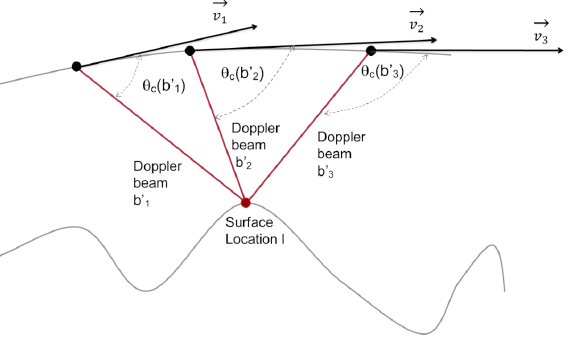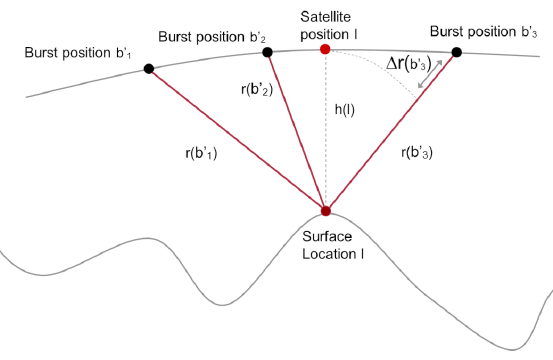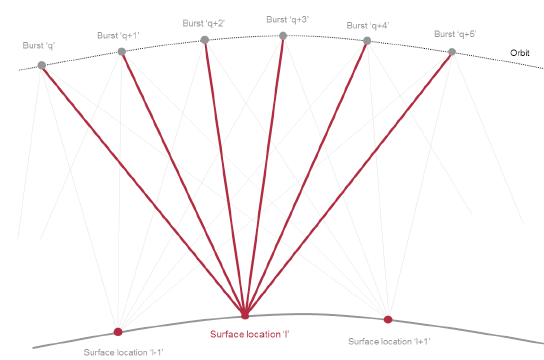## Level-1 SAR_Ku processing chain

See S3 SRAL Algorithms Technical Baseline Document for further information about S3 Altimetry algorithms.

The main algorithms of the Level-1 SAR_Ku chain are:

• Determine surface type

This algorithm computes the surface type ("open ocean or semi-enclosed seas", "enclosed seas or lakes", "continental ice" or "land") determining the position of a "land-sea mask" Auxiliary Data File nearest to the geolocated measurement. The latitude and longitude resolution of this land-sea mask is 2 minutes.

• Compute tracker ranges corrected for USO frequency drift

This algorithm computes the USO correction from an Auxiliary Data File called "USO file" and this correction is applied to the tracker range. The "USO file" provides the real USO frequency drift measured on-board wrt the USO frequency nominal value. This algorithm also computes the tracker range rate converted into distance versus time.

• Compute tracker ranges corrected for internal path correction

This algorithm computes the internal path correction from an Auxiliary Data File called "CAL1 LTM file" and this correction is applied to the tracker range. The "CAL1 LTM file" provides the internal path delay measured on-board thanks to the CAL1 calibration mode, which measures the difference of travel between the transmission and the reference lines within the altimeter. This algorithm also computes and applies the instrumental delay correction measured on-ground, due to the distance between the duplexer and the antenna reference point.

• Correct the AGC for instrumental errors

This algorithm computes the Automatic Gain Control (AGC) instrumental correction and applies this correction to the AGC. The AGC instrumental correction is computed taking into account the real gain value applied on-board and stored as a matrix table on an Auxiliary Data File called "characterisation file".

• Correct and apply power & phase corrections

This algorithm computes and applies to each burst the phase and power variations within all the echoes of every burst. These phase and power corrections are measured on-board through a sequence of calibration echoes in CAL1 calibration mode.

• Correct the waveforms.

On-board, there is a calibration mode called CAL2 that is able to compute the Gain Profile Range Window (GPRW) that provides the information of the attenuation of the samples of the Level . The GPRW accounts for several instrumental effects (e.g. intermediate frequency filters gain response) that have an impact on the Level 0 waveforms power. This algorithm corrects these Level-0 waveforms by the GPRW instrumental effects.

• Compute surface locations

In the SAR_Ku processing chain, the output measurements are referenced to surface locations along the satellite track. These surface locations correspond with the intersection of the Doppler beams with an estimation of the surface elevations. These surface locations are used along all L1 SAR_Ku processing.

• Determine Doppler beams direction

This algorithm determines the angular spacing between the instantaneous zero Doppler plane and the lines defined by the burst centre and the reference surface locations "observed" within the burst sequence.

• Doppler beams generation

This algorithm generates the Doppler beams in the frequency domain. Each burst of pulse-limited time domain echoes are transformed into the frequency domain using an FFT (Fast Fourier Transform) in the along track direction.

• Compute and apply Doppler correction

This algorithm computes and applied the Doppler correction to the tracker ranges. This correction is needed to remove the echoes frequency shifts due to sensor-target velocity. The Doppler correction is computed and applied in the frequency domain to each Doppler beam. This correction is a function of the emitted frequency, the pulse emitted duration, the satellite velocity of the beams, the emitted bandwidth and the sign of the slope of the transmitted chirp.Figure 1: Doppler shift effect (Credit: isardSAT)

• Compute and apply slant range corrections

This algorithm computes the slant corrections (both fine and coarse) that correct the range-migration due to the motion of the sensor along the orbit.Figure 2: Slant range correction (Credit: isardSAT)

• Range compression

This algorithm performs a range compression of the waveform that is the conversion of each Doppler processed burst of pulse-width time domain echoes to the frequency domain.

• Tracker alignment correction

This algorithm corrects the azimuth processed echo stack for on-board tracker variation. It means that for each surface location, the waveforms are aligned before multi-looking.

• Doppler beams stack & multi-looking

This algorithm computes the stacked Doppler beams (I2+Q2 power waveforms) through the non-coherent summation of all the beams corresponding to each surface location.Figure 3: Doppler beams stack (Credit: isardSAT)

• Compute sigma0 scaling factor

This algorithm computes the sigma0 scaling factor that is used at Level2 to determine the backscatter coefficients from the retracked amplitudes. The sigma0 scaling factor accounts for all power attenuations and gains which have an impact on the signal received on-board.